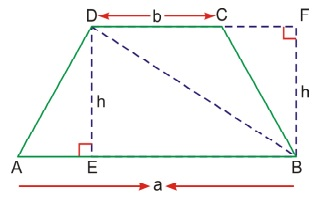# Area of Trapezium

Area of a trapezium = (half the sum of parallel sides) × height

Area of trapezium = Area of ΔABD + Area of ΔBCD= (1/2 × a × h) + (1/2 × b × h)

= 1/2 × (a + b) × h

Area of a trapezium = (half the sum of parallel sides) × height

Example 1: Find the area of a trapezium whose parallel side are 20 cm & 12 cm and the distance between parallel lines is 3 cm.

Area of a trapezium = 1/2 × sum of parallel side × height

= 1/2 × (20 + 12) × 3

= 1/2 × 32 × 3

= 48 cm2